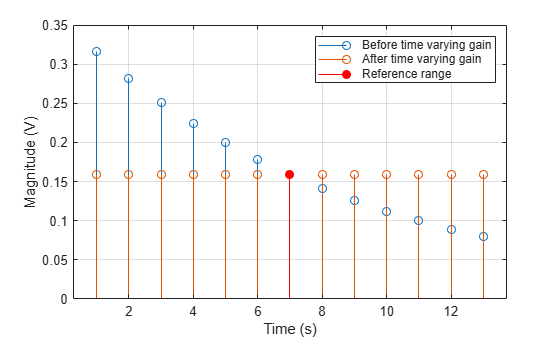phased.TimeVaryingGain

Time varying gain control

Description

The TimeVaryingGain object applies a time varying gain to input signals. Time varying gain (TVG) is sometimes called automatic gain control (AGC).

To apply the time varying gain to the signal:

1. Define and set up your time varying gain controller. See Construction.

2. Call step to apply the time varying gain according to the properties of phased.TimeVaryingGain. The behavior of step is specific to each object in the toolbox.

Note

Starting in R2016b, instead of using the step method to perform the operation defined by the System object™, you can call the object with arguments, as if it were a function. For example, y = step(obj,x) and y = obj(x) perform equivalent operations.

Construction

H = phased.TimeVaryingGain creates a time varying gain control System object, H. The object applies a time varying gain to the input signal to compensate for the signal power loss due to the range.

H = phased.TimeVaryingGain(Name,Value) creates an object, H, with each specified property Name set to the specified Value. You can specify additional name-value pair arguments in any order as (Name1,Value1,...,NameN,ValueN).

Properties

 RangeLossSource Source of range losses Specify the source of range losses as either 'Property' or 'Input port'. When you specify RangeLossSource as 'Property', the range loss for each sample is set in the RangeLoss property. When you specify the RangeLossSource as 'Input port', the range losses are specified using an input argument to the step method. Default: 'Property' RangeLoss Loss at each input sample range Specify the loss due to range as a vector — elements correspond to the samples in the input signal. Units are in dB. This property can have single or double precision. Default: 0 ReferenceLoss Loss at reference range Specify the loss at a given reference range as a scalar. Units are in dB. This property can have single or double precision. Default: 0

Methods

 step Apply time varying gains to input signal
Common to All System Objects
release

Allow System object property value changes

Examples

collapse all

Apply time varying gain to a signal to compensate for signal power loss due to range.

First, create a signal with range loss. Set the reference loss to 16 dB.

rngloss = 10:22;
refloss = 16;
t = (1:length(rngloss))';
x = 1./db2mag(rngloss(:));

Then add gain to compensate for range loss.

gain = phased.TimeVaryingGain('RangeLoss',rngloss,'ReferenceLoss',refloss);
y = gain(x);

Plot the signal with loss and the compensated signal.

tref = find(rngloss==refloss);
stem([t t],[abs(x) abs(y)])
hold on
stem(tref,x(tref),'filled','r')
xlabel('Time (s)'); ylabel('Magnitude (V)')
grid on
legend('Before time varying gain','After time varying gain',...
'Reference range')expand all

References

 Edde, B. Radar: Principles, Technology, Applications. Englewood Cliffs, NJ: Prentice Hall, 1993.

 Skolnik, M. Introduction to Radar Systems, 3rd Ed. New York: McGraw-Hill, 2001.